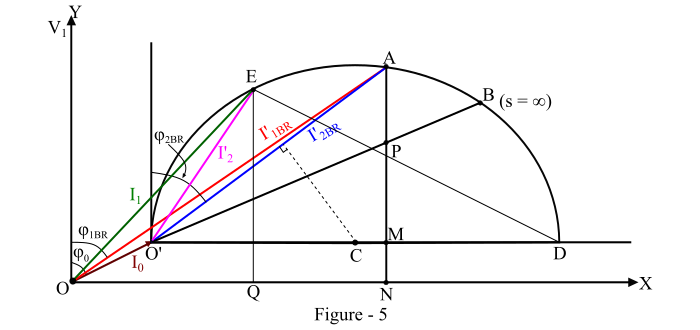# Construction of Circle Diagram of Induction Motor

Digital ElectronicsElectronElectronics & Electrical

## Circle Diagram of Induction Motor

The circle diagram is a graphical representation of the performances of an induction motor. It is very useful to study the performance of an induction motor under all operating conditions. The construction of the circle diagram of an induction motor is based on the equivalent circuit of the motor which is shown in Figure-1.By applying KCL in the equivalent circuit, we can write,

$$\mathrm{𝐼_1 = 𝐼_0 + 𝐼′_2 … (1)}$$If the phase voltage V1 is taken along the vertical axis of OY as shown in Figure-2. Then, the no-load current (I0) lags behind the voltage V1 by an angle ϕ0. Where, ϕ0 is the no-load power factor angle and is of the order of 60°-80° because to produce the required flux per pole in the air gap of the machine, a large magnetising current is needed.

Now, at no-load, the slip s = 0, then

$$\mathrm{\frac{𝑅_2}{𝑠}= ∞ \:𝑖.𝑒.\: open\: circuited \:at \;no − load}$$

$$\mathrm{\therefore 𝐼_0 =\frac{𝑉_1}{𝑍_{𝑁𝐿}}… (2)}$$

Where,

$$\mathrm{𝑍_{𝑁𝐿} = (𝑅_0 \:||\: 𝑗𝑋_0) = No \:load \:impedance}$$

Here, all the rotational losses are taken into account by R0. Then, the no-load losses are given by,>

$$\mathrm{𝑃_0 = 𝑉_1𝐼_0 cos \varphi_0 … (3)}$$

The rotor current referred to the stator side is given by,

$$\mathrm{𝐼′_2 =\frac{𝑉_1}{𝑍′_{𝑒1}}=\frac{𝑉_1}{\sqrt{(𝑅_1 +\frac{𝑅′_2}{𝑠})^2+ (𝑋_1 + 𝑋′_2)^2}}… (4)}$$

This rotor current I′2 lags behind the voltage V1 by the impedance angle ϕ.

Refer Figure-3,$$\mathrm{sin\:\varphi =\frac{𝑋_1 + 𝑋′_2}{\sqrt{(𝑅_1 +\frac{𝑅′_2}{𝑠})^2+ (𝑋_1 + 𝑋′_2)^2}}… (5)}$$

Hence, by combining the eqns. (4) & (5), we get,

$$\mathrm{𝐼′_2 =\frac{𝑉_1}{𝑋_1 + 𝑋′_2}sin \varphi; … (6)}$$

Eqn. (6) is in the form of 𝑟 = 𝑎 sin θ, which represents a circle with diameter a. Therefore, the locus of current (𝐼′2) is a circle whose diameter is

$$\mathrm{diameter =\frac{𝑉_1}{𝑋_1 + 𝑋′_2}}$$

It is shown in Figure-4.The radius of the circle is,

$$\mathrm{O′C =\frac{𝑉_1}{2(𝑋_1 + 𝑋′_2)}}$$

From eqn. (1), it is clear that the stator current I1 is found by combining the results shown in Figures-2, 3, and 4. Then, the resulting figure obtained is shown in Figure-5 which is known as the circle diagram of the induction motor.

## Construction of Circle Diagram of Induction Motor

The data required to draw the circle diagram of an induction motor are −

• Stator phase voltage,
• $$\mathrm{𝑉_1 =\frac{𝑉_𝐿}{\sqrt{3}}}$$

• No-load current (I0) of the motor
• No-load power factor (cos ϕ0)
• Stator resistance per phase (R1)
• Blocked rotor current and the power factor.

The procedure for drawing the circle diagram of the induction motor is given as follows −

•  Step 1 – Take the stator voltage phasor (V1) along the y-axis.
•  Step 2 – Choose a convenient scale for the current. With origin O, draw a line segment OO’ = I0 at an angle ϕ0 with voltage phasor V1.
•  Step 3 – Draw a line OQN perpendicular to voltage (V1). Also, draw a line O’D perpendicular to voltage V1.
•  Step 4 – From origin O draw a line of length equal to the blocked rotor current (I1BR) to the same scale as that of I0. This line lags behind the voltage phasor V1 by an angle equal to the blocked rotor power factor angle (&varphi_{1BR}).
•  Step 5 – Join O’A and measure its magnitude in amperes. The magnitude of the line O’A is the value of current (I’2BR).
•  Step 6 – From the point A, draw a line AMN which is parallel to voltage phasor V1. This line is perpendicular to O’D and ON.
•  Step 7 – Calculate the value of MP as,

$$\mathrm{MP =\frac{𝐼′_{2𝐵𝑅}^{2}𝑅_1}{𝑉_1}}$$

And locate point P. Then, join O’P and extend it to meet the circle at point B. It is to be noted that slip s is infinity at the point B.
•  Step 8 – Now, draw the perpendicular bisector of the chord O’A. This bisector will pass through the centre of the circle C. Therefore, with radius CD’ or CD, draw the circle, which is the circle diagram of the induction motor (see Figure-5).